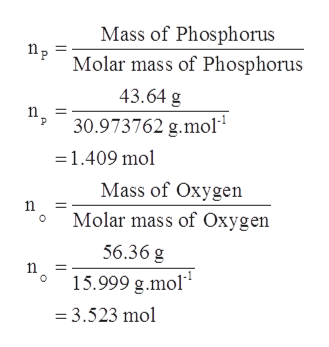# Determine the empirical and molecular formulas of a compound that contains 56.36% O and 43.64% P and has a molar mass of 284 g/mol.

Question
10 views

Determine the empirical and molecular formulas of a compound that contains 56.36% O and 43.64% P and has a molar mass of 284 g/mol.

check_circle

Step 1

It is given that:

Amount of oxygen (O) in compound = 56.36 %

Amount of Phosphorus (P) in compound = 43.64 %

Molar mass of the compound = 284 g/mol

Step 2

Chemical formula or molecular formula represents the type of atoms and number of atoms present in a molecule using numerical subscripts and atomic symbol.

The representation of atoms of a compound in simple whole number ratio is known as empirical formula. The rules for determining empirical formula is given as follows:

• Determine the mass of elements in given compound.
• Calculate number of moles using molar mass of each compound or element.
• Divide each number of mole by smallest number of mole value calculated in second step.
• Round the value calculated in step 3 to nearest whole number.
Step 3

1. Assuming the amount of phosphorus and oxygen is given mass by mass.

Thus, mass of phosphorus = 56.36 g

Mass of oxygen ...help_outlineImage TranscriptioncloseMass of Phosphorus np Molar mass of Phosphorus 43.64 g п 3 30.973762 g.mol n, =1.409 mol Mass of Oxygen Molar mass of Oxygen 56.36 g 15.999 g.mol = 3.523 mol fullscreen

### Want to see the full answer?

See Solution

#### Want to see this answer and more?

Solutions are written by subject experts who are available 24/7. Questions are typically answered within 1 hour.*

See Solution
*Response times may vary by subject and question.
Tagged in

### General Chemistry